McDonald, J. D., and Kerekes, R. (2017). "Pragmatic mathematical models of wet pressing in papermaking," BioResources, 12(4), 9520-9537.

#### Abstract

This paper compares mathematical models developed over the years to estimate the moisture content of paper after wet pressing on paper machines. Models that assume all loading pressure to act upon water are discussed in detail. In contrast, most conventional models assume that pressure splits between a hydraulic component causing water removal and a structural component supported by the fibres. This assumption is shown to be questionable in light of theoretical considerations and experimental evidence. Despite their simpler nature, models based on hydraulic forces alone are often better able to account for experimental observations. The Decreasing Permeability (DP) model is the most complete model of this form and includes all major variables affecting pressing on paper machines, thereby enabling evaluation of key factors such as the relative importance of dewatering under flow-controlled and pressure-controlled conditions together with rewetting.

Pragmatic Mathematical Models of Wet Pressing in Papermaking

J. David McDonald,a,* and Richard J. Kerekes b

This paper compares mathematical models developed over the years to estimate the moisture content of paper after wet pressing on paper machines. Models that assume all loading pressure to act upon water are discussed in detail. In contrast, most conventional models assume that pressure splits between a hydraulic component causing water removal and a structural component supported by the fibres. This assumption is shown to be questionable in light of theoretical considerations and experimental evidence. Despite their simpler nature, models based on hydraulic forces alone are often better able to account for experimental observations. The Decreasing Permeability (DP) model is the most complete model of this form and includes all major variables affecting pressing on paper machines, thereby enabling evaluation of key factors such as the relative importance of dewatering under flow-controlled and pressure-controlled conditions together with rewetting.

Keywords: Water removal; Paper machines; Mathematical models; Wet pressing

Contact information: a: JDMcD CONSULTING INC., 97 Rue Kerr, Vaudreuil-Dorion, Quebec, Canada J7V 0G1; b: University of British Columbia,2385 East Mall, Vancouver, British Columbia, Canada V6T 1Z4; *Corresponding author:mcdonald.jdavid@gmail.com

INTRODUCTION

Wet pressing is a key unit operation in papermaking. Accordingly, it is desirable to have mathematical models to predict the extent of water removal caused by wet pressing, in particular models that are relatively easy to use by practitioners. Over the years, numerous attempts have been made to accomplish this, but these have generally not been successful in meeting this objective. A review of wet pressing research (Macgregor 1989) and pressing models (El-Hosseiny 1991) concluded that past efforts had limitations that precluded their use as design or optimization tools. This paper examines the reasons why and reviews progress that has been made in the past 25 years.

THE WAHLSTROM MODEL

In pioneering work, Wahlstrom laid the foundation upon which almost all pressing models have been built (Wahlstrom 1969). This model is based on the concept that the total pressure applied by the press (σT) is opposed by parallel pressures: “hydraulic pressure” (σH) that causes water removal and “structural pressure” (σS) that is supported by the fibres. As water is expelled from the web, hydraulic pressure diminishes and structural pressure increases, eventually coming to an equilibrium wherein the entire pressure is borne by the structural pressure. This is the Terzaghi principle of pressing fluid from a suspension of solid particles (Terzaghi et al. 1996), shown in the equation form below: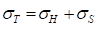(1)

Later work (Carlsson et al. 1977) found that significant water was expelled from within fibres, even in the early stages of pressing. To account for this, Wahlstrom (1990) added a third pressure term (σF) for the hydraulic pressure within fibres: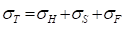(2)

Somewhat surprisingly, this parallel pressure model has been accepted uncritically over the years. It is an enormously appealing concept. Nevertheless, MacGregor concluded that “although this model agrees qualitatively with observations, it has not been substantiated with measurements” (MacGregor 1989). Close examination of its implications reveals serious flaws.

Many scientific laws (electricity, heat transfer, fluid mechanics) are based on flow being proportional to a driving force divided by resistance. Consequently, absence of flow is due to either zero driving force (e.g. power failure) or infinite resistance (e.g. light switched off). The Wahlstrom model is based entirely on driving force (pressure). Accordingly, the implication at equilibrium is that no pressure at all is exerted on any water in the web. Otherwise, the water would flow. This seems scarcely credible given that more water than fibre remains in the web at the end of wet pressing. Furthermore, wet pulp fibres are easily collapsible and therefore unlikely to have the structural integrity to entirely shield water from pressure.

The flaw in the Wahlstrom model is evident in the most common physical analogy used to depict it—the Kelvin-Voigt model shown in Fig. 1. This model is a mechanical analogy of the pressing process. In it, force acts upon a spring and dashpot in parallel.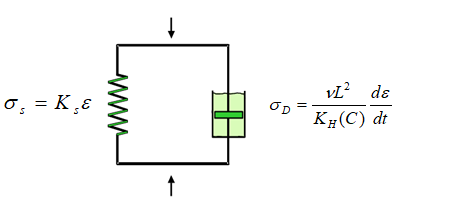Fig. 1. Kelvin-Voigt Model with pressures exerted by the spring for a strain (ε) and the dashpot for a change in strain (dε/dt), as derived from Darcy’s lawThe initial high rate of compression mirrors the initial high speed of water removal. Equilibrium is ultimately reached when the spring supports all of the pressure. However appealing this model may be, it does not predict one of the most widely observed experimental findings in wet pressing, that the product of pressure and time, the ‘press impulse’ describes dewatering very well in many cases (Busker and Cronin 1984; Kerekes and McDonald 1991). The failure of the Kelvin model to predict this importance of press impulse is evident in the equation that describes it (Caulfield et al. 1982; Wegner et al. 1983; Young et al. 1983; Caulfield et al. 1986), as shown below: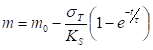(3)

Here m0 is the moisture ratio before the press (expressed as weight of water divided by dry weight of fibre), m is the moisture after the press, is time, Ks is the modulus of the structure, and τ is a time constant characteristic of the material. In this equation, pressure and time are separated by an exponential term and can only come together as a product in the trivial case of infinitely small dewatering. This observation alone casts doubt on the model.

Other problems with this model lie with the constants. The response of the “structure” is represented by a spring that resists linearly with pressure. In reality, the response of a fibre matt is highly non-linear, best represented as a power function of applied pressure. The time constant is another issue. Carlsson used Darcy’s law to derive a relationship for the time constant (Carlsson et al. 1983),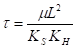(4)

where μ is the viscosity of water, KH is the Darcy permeability, and L is the thickness of the fibre mat. Although these give a reasonable fit to pressing data over a limited range of conditions (Caulfield et al. 1982; Wegner et al. 1983; Young et al. 1983; Carlsson et al. 1983; Caulfield et al. 1986) they are not really constants but depend upon the state of pressing. The thickness (L), permeability (KH), and fibre mat spring constant (Ks) are non-linear functions of the degree of dewatering. Hence, this model cannot be used to predict water removal for different conditions.

A further shortcoming of the Wahlstrom picture lies in accounting for how water is expelled from individual fibres. How does pressure on a fibre split into a true structural pressure and a pressure that causes water removal? Unless fibres are absolutely rigid, which is not the case for wet pulp fibres (Tam Doo and Kerekes 1982; Dunford and Wild 2002; McDonald et al.2014b), pressure exerted upon them acts through their walls to water within them, and on fibres below them. Water is not shielded from pressure by the solid structure. Thus, if water is not expelled from a fibrous web, the likely cause is not absence of pressure, but effectively infinite resistance to flow in the form of closed-off pathways (Maloney et al. 1997). In short, pressure is pressure, whether exerted upon water directly or through fibre walls. Water under pressure from any source will flow unless it faces infinite resistance.

Although equations based on Wahlstrom’s picture have been constructed and solved numerically (Roux and Vincent 1991; Kataya et al. 1992; Jönsson and Jönsson 1992a,b; Kataya et al. 1995; Fitt et al. 2002; Gustasfsson et al. 2003; Lobosco 2004; Bežanović et al. 2006, 2007; Kataya. 2009; Printsypar 2012; Iliev et al. 2012, 2013) the necessary material parameters are either difficult or impossible to measure. For example, the “structural” integrity of the fibres depends on their water content. Web permeability is a combination of permeability between fibres, in lumens, and within fibre walls.

In summary, the parallel pressure model of wet pressing paper, though enormously appealing, is a flawed concept for pressing wet pulp fibres. In 1991, 30 years after its first publication, the authors concluded that this conventional model was a major impediment to progress in modeling wet pressing in papermaking (Kerekes and McDonald 1991). We undertook to derive an alternative model based upon driving force and resistance. In doing so, we considered the various factors affecting this problem. These are reviewed below along with the work of others who followed a path similar to ours, that is, one not based on the concept of parallel pressures.

Regression Models

Attempts to model the pressing process have taken several forms. The simplest approach is to use multiple-regression fitting of laboratory, pilot, or commercial data to parameters at operating conditions that are expected to affect water removal (DeCrosta and Plaisted 1978; DeCrosta 1982). This approach has been used to evaluate the relative importance of different pressing parameters. The equation is created by multiplying the parameters raised to a power (DeCrosta and Plaisted 1978),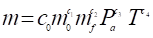(5)

where m0 is the web moisture before the press nip, mf is the felt moisture before the press nip, Pa is the average nip pressure (kPa), and is the nip residence time (ms).

The coefficients (c0c1c2c3, and c4) were determined by multiple linear regression after taking the logarithm of Eq. 5. This approach can identify the relative importance of the parameters and can be used to give approximate answers within the range of the data set.

In later work (DeCrosta 1982), the resistivity of the felt, Rf, was included,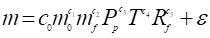(6)

where Pis the peak pressure (MPa), Rf is the relative resistivity of the felt, and ε is for unaccounted variables.

Linear regression of data from a pilot press showed that 87% of the variation could be explained and was dominated by the ingoing moisture (60%) and the peak pressure (24%) (DeCrosta 1982). Felt moisture and resistivity have a negligible effect.

Another approach used linear- multiple regression with second order terms (Cox and Robertson 1981):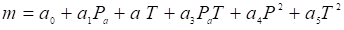(7)

As with Eq. 5, the advantage of curve-fitting is that an exact understanding of the mechanism of dewatering is not necessary. On the other-hand, for this complicated, non-linear problem, it is difficult to separate interactions between parameters and predict behavior beyond the collected data. This approach had limited success and should be used with caution because change in testing conditions could lead to erroneous predictions.

Flow through Porous Media

Dewatering of paper, board, pulp, or tissue by pressing is a problem of water flow from porous media because both the web and felt are porous structures. The science of flow through porous media has been extensively developed, particularly for groundwater flow through soil and rock and, more recently for oil and gas extraction (Scheidegger 1960; Bear 1988). However, there are several complications when applying these equations to pressing. The geometry of a fibrous web is not regular and must be determined by an independent measurement. Furthermore, the geometry of the fibres and consequent flow path lengths and resistance per unit length in the web continuously change as the web is compressed and water is removed. Finally, water does not flow through the web from an external source. It flows from within the web, from the spaces between fibres and from within fibres themselves. The flow from the lumens and cell walls of the fibres is significant and represents the most difficult water to remove.

Darcy’s Law

The most common causal approach to modeling wet pressing is by applying equations that have been developed to describe fluid flow in porous media. The simplest and most intuitive of these is Darcy’s law (Fig. 2) (Scheidegger 1960; Bear 1988). This is an empirical equation originally developed to relate fluid velocity to the pressure across a packed bed of material,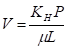(8)

where V is the fluid velocity, is pressure, KH is permeability, is fluid viscosity, and L is the depth of the packed bed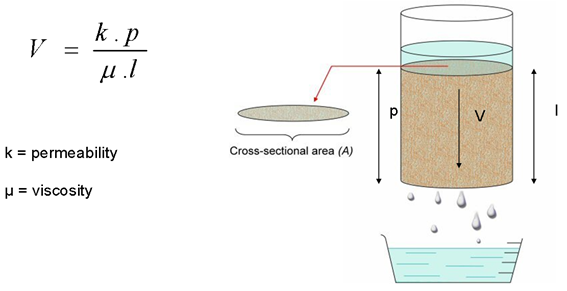Fig. 2. Darcy’s Law

Much past work has focused on determining permeability KH from fundamental parameters. The most rigorous approach is based on the fundamental equations for fluid flow, namely the Navier-Stokes equation with appropriate boundary conditions for the porous medium. The resulting equations are difficult to solve. Solutions exist only for structures with simple and well-defined geometry, for example a series of parallel tubes with specified radii and lengths and lead to the Poiseuille equation.

In typical applications of the Darcy equation, the depth of the material is a constant, and it is easily measured in the case of incompressible materials. Temperature dependence is incorporated in the fluid viscosity. The permeability for a specific material is an empirical constant that can be calculated by measuring the fluid velocity and the resulting pressure across the bed.

Kozeny-Carman Equation

For more complex porous media, the Kozeny-Carman equation (9) is often used in place of Darcy’s law (Scheidegger 1960; Bear 1988). Here permeability, KH, is defined in terms of measurable variables such void volume (volume not occupied by solid material) and the specific surface of the solid material. There is an additional factor, the Kozeny constant, Kc, to account for tortuosity or the increased path length that the fluid will take in traversing the porous media. One form of the Kozeny-Carman equation is,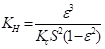(9)

where ε is the void volume (volume occupied by water) and S is the specific surface area.

The validity of the Kozeny-Carman equation for characterizing porous media has been questioned, in particular for mats of fibrous material (Scheidegger 1960). For many materials, shape and dimensions of the void volume are more important than the size of the void volume. The central issue in using the K-C equation for wet pressing is an appropriate value of permeability KH. Indeed, this has been the Achilles heel of many pressing models.

Permeability in Wet Pressing

Various approaches have been taken to determine the permeability KH for wet pressing. A common approach is flow-through experiments in packed beds of well-defined particles or engineered structures with known geometry. Such experiments can also be carried out on pulp mats. In a typical experiment, water is forced through a fibrous mat, which is compressed to different levels. This measures the flow of water between the fibres. Such measurements have been employed to evaluate the early stages of pressing (McDonald and Kerekes 1991). However, this approach does not account for the flow of water from the fibres, which is significant for a highly pressed sheet (Scallan 1966; Carlsson et al. 1977; Laivins and Scallan 1994).

Most important, there is no single value of KH during the course of wet pressing. As moisture content decreases, fibre- level flow dominates, and therefore permeability decreases. To reflect this, permeability would have to be measured in specially-designed laboratory experiments as a function of the degree of dewatering and compaction. This becomes difficult for flow from fibre lumens, and even more so for flow from cell walls. In the latter case, solute exclusion or mercury intrusion might provide information on flow paths and sizes. New techniques such as X-ray tomography (Kataya 2009; Koivu et al. 2009a,b, 2010) can be used to precisely map the void volume between fibres. However, these do not yet have the resolution to discern the structure of the fibre wall.

Attempts to account for decreasing permeability have taken various forms. In the Darcy equation, KH has been divided by the radius of the fibres (a) in a matt and expressed as a function of the solid fraction (ϕ) for a wide range of materials and scales (Jackson and James 1986):

KH/a2 = f(ϕ) (10)

However, this does not necessarily apply to flow from the fibre wall and lumens.

Similar approaches have been taken for the K-C equation. Various functions involving the solid fraction (ϕ) to describe permeability (Jackson and James 1986) or different forms of the Kozeny-Carman equations for fibrous materials have been used. However, these introduce parameters that are difficult or impossible to determine by independent means or they become adjustable parameters that give the expected result (Scheidegger 1960).

In summary, limitations exist in modeling permeability in wet pressing. Given the desire to have a useable equation to predict water removal, the need is to select or develop an approach having the fewest possible assumptions, each of which is physically sound. One such approach is to express permeability as a function of moisture content and build this into the dewatering model equation, i.e. Darcy’s law or the Kozeny-Carman equation. If the assumptions and model are reasonably sound, then the parameters obtained by fitting the model to pressing data will have predictive capabilities beyond the cases employed for the curve fitting. We now consider approaches to accomplish this.

Models of Wet Pressing

A typical approach is to apply Darcy’s law (8) or the Kozeny-Carman equation (9) with the continuity equation below,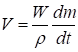or V = dC/dt… (11)

where W is the basis weight and ρ is the density of water.

This assumes that pressure applied by the press nip is entirely supported by water, the fibre mat is saturated, the felt backing roll is vented, and the flow resistance of the felt is negligible compared to that of the web. Additional equations are necessary to relate permeability and matt thickness to consistency or moisture ratio. Doing so is complex because permeability of the fibre matt and its thickness decreases as water is removed.

Campbell’s Approach (1947)

Campbell assumed that load in a press nip was entirely supported by hydraulic pressure acting uniformly through the sheet thickness (Campbell 1947). His argument was based on the large amount of water in the web and the short dwell time in the nip. Using Darcy’s law and assuming that the rate in change of web thickness is proportional to the flow of water (continuity equation),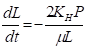(12)

where P is the applied pressure, is the web thickness, KH is Darcy permeability, and μ is the viscosity of water

Applying the Kozeny-Carman equation and assuming that the Kozeny coefficient which takes into account that the path length is greater than L, (5 for non-orientated masses) the Darcy permeability can be expressed as,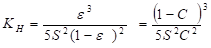(13)

where ε is the void volume (volume occupied by water), S is the specific surface area, and C is the consistency (1-ε).

This is a nonlinear relationship between consistency and permeability, showing that permeability decreases as web consistency increases. Substituting Eq. 13 into Eq. 12 and integrating gives,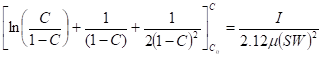(14)

where is the consistency after the nip, C0 is the consistency before the nip, is the basis weight, S is the specific surface area, is the viscosity of water, and I is the press impulse, where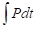(15)

This non-linear equation (14) includes the effect of press impulse, basis weight, and temperature (through viscosity). However, solving this equation for consistency after pressing is difficult because of the awkward term on the left-hand side of Eq. 14. Also, specific surface is not likely to be independent of consistency and the choice of Kozeny constant is questionable. However, considering the early date of publication, this paper was path-breaking.

Luey’s Approach (1979)

Luey used Darcy’s law and Poiseuille’s equation to derive the following equation (Luey 1979),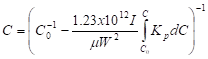(16)

where Kp is a pressing permeability constant, which is defined as,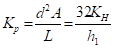(17)

where d is the pore diameter, A is the pore cross-sectional area, L is the pore length, and h1 is the web thickness for 1 g fibres/cm.

The pressing permeability constant can be represented by a cubic equation: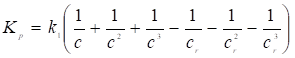(18)

Here Cr is the critical consistency at which all free water, defined as water not chemically bound to the fibres, has been removed and above which there is no further change in permeability.

This model introduced two important concepts: a non-linear relationship between permeability and consistency, and a critical consistency at which no further dewatering is possible. Measurements of handsheets and pressed samples from paper machines with flow through cell (Robertson and Mason 1949) were fit to Eq. 18 to determine k1, a furnish-dependent coefficient and cr, the critical consistency. The permeability/consistency relationship (16) can be solved iteratively for the consistency, c, after pressing. However, this approach is limited, not by the model equation, but by the method to determine the permeability. The measurements with the flow-through cell do not account for the flow from the cell wall of the fibres. Consequently, there were discrepancies between the critical consistency determined by this method and multiple passes through a press nip.

Gudehus’ Model (1988)

As described in a review of pressing models (El-Hosseiny 1991), Gudehus (Gudehus 1988a,b,c) developed a water removal equation for pressing by modeling paper as a series of circular tubes that, as water is removed, shrink in diameter but retain their length by bending (Gudehus 1988b),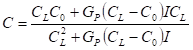(19)

where C is the consistency after pressing, C0 is the consistency before pressing, CL is the limiting consistency (pressed consistency at constant pressure for infinite time), and I is the press impulse. The parameter GP is a pressing factor that accounts for temperature and furnish, which is defined as,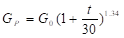(20)

where t is the temperature of the web in ̊C and G0 is defined as: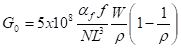(21)

In Eq. 21, N is the number of tubes per unit area, L is the length of the tubes, αf is the percent contact area of the felt, is a structure factor to account for tube bending, W is the basis weight, and ρ is the density of water.

Gudehus was not able to relate f, N, and L to the properties of the web. Instead, he calculated GP using pilot and laboratory pressing data from the literature. Using selected values of GPand CL he calculated pressed solids for a number of different presses and press configurations (Gudehus 1988c).

This model has the potential to calculate the pressed solids for the flow-controlled regime although comparisons were not made to commercial measurements in his publications. Equations give the range of conditions where this equation is valid (Gudehus 1988c).

The model uses easily measured parameters such as press impulse, temperature, and ingoing consistency and two furnish parameters: the limiting consistency, CL , which can be measured in the laboratory and the pressing factor, GP, which can be determined from pilot or commercial pressing data. The model accounts for the effect of temperature through viscosity and decreasing permeability of the web with water removal through the pressing factor GP.

The Limiting Consistency Model (1994)

Limiting consistency (CL) is defined as the maximum consistency that would be reached by applying a pressure (P) for infinite time (Clos et al. 1994). Assuming that the applied pressure is solely supported by water, applying the Kozeny-Carman equation with the limit imposed by CL gives: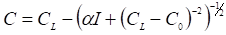(22)

where C is the consistency after pressing, C0 is the consistency before pressing, CL is the limiting or maximum consistency, is the press impulse, and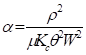(23)

where ρ is the density of water, μ is the viscosity of water, Kc is the Kozeny constant, is the specific surface area, and is the basis weight.

These equations have the potential to quantitatively represent the effect of press impulse, basis weight, specific surface and temperature (viscosity) on pressed solids. The model was fit to data from a pilot press (Caulfield et al. 1982) to determine the two parameters: α and CL. The authors concluded that CL was a function of applied pressure and specific surface area (degree of refining). Although the fit was good with a normalized error of 1.4%, parameter α changed with press nip load and CL changed with inlet consistency for a given furnish. Each pressing condition requires a unique α, which means that this model cannot be used to predict or calculate new pressing conditions. The limiting consistency (CL) should only depend on press nip pressure. Clearly this model is a poor match to the physical situation in its present form.

Further problems with this approach are related to several assumptions made in the derivation of this equation. The first was that the flow path, L, could be treated as a constant by assuming that the path length was like a spring which would not change under compression. This might be true if all the water was contained between the fibres. However, in the later stages of pressing, when a greater portion of the water flows from the fibre walls, this is not the case. Similarly, the specific surface Θ, which is embedded within the parameter α will depend on consistency as water flows from smaller and smaller pores in the cell wall. The authors claimed that both CL and α could be calculated from readily measurable variables but did not show how this could be done.

Holstege Model (1998)

Holstege (1998) used Luey’s equations (Luey 1979) as a starting point but introduced the concept of critical dryness limit (CL) , defined as the maximum consistency that can be obtained in a specific press nip when the average nip pressure is applied for infinite time.

Measurements in a platen press were fit to the following power function:

C= aPan (24)

Relationships for pore diameter, pore length, and pore drainage area can be written, respectively, as,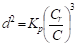(24a)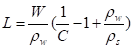(24b)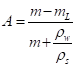(24c)

Using 24a, 24b and 24c to solve for Kp in Eq. 17 and substituting into Eq. 16 and integrating gives: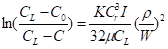or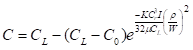(25)

Assuming a constant value for rewet and using a value for CL from static measurements in a platen press, pressed solids after the third press on a commercial corrugating medium machine at five basis weights from 120 to 200 g/m2 were fit to equation 25 to determine the furnish dependent parameter. During the course of these trials, the calculated value ofdecreased and this was attributed to changes in furnish characteristics.

This approach has several notable features, namely an independent measurement of the limiting consistency (CL) and determination of a single furnish dependent parameter () from pressing data to characterize the permeability of flow from the web. This avoids the problem with laboratory measurements to measure permeability, which, as stated earlier, only measure flow between fibres and exclude flow from fibres. Calculations of the change in pressed solids with temperature based on the change in viscosity gave 1%/10 oC, which is in accord with empirical observations. Further work would need to be done to determine whetheris truly representative of the furnish and constant with respect to changes in press impulse (nip load and speed).

Decreasing Permeability Model (1991-2017)

In the early 1990’s, the authors began studies on a model of wet pressing based upon pressure acting on a wet web in which permeability decreased as water was expelled from it (Kerekes and McDonald 1991; McDonald and Kerekes 1991). The model was based on the simplest assumptions that could be justified, namely that pressure acts on water throughout the web, whether directly or through fibre walls, and that permeability and flow path length have a non-linear dependence on the moisture content of the web,

KH= Bmb and L = Cmc (26)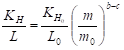(27)

whereand L0 are, respectively, the permeability and thickness of the web before the press nip.

An early version of the DP model was derived from Darcy’s law for the flow-controlled regime by assuming that the moisture was above the equilibrium moisture and rewet was negligible (Kerekes and McDonald 1991). The model was shown to give good fits to commercial paper machine survey data (McDonald and Kerekes 1991),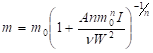(28)

where A=B/C is the specific permeability (g/m), is the press impulse (kPa.s), n = b-c-1 is the compressibility factor, W is the basis weight (kg/m2), and ν is the kinematic viscosity (m2/s).

To account for rewet (R), in 1995 the authors added an additional term (McDonald and Kerekes 1995; McDonald et al. 2000):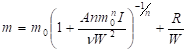(29)

The concept of rewet in wet pressing is very controversial. Some believe that it is water sucked back into the web from the felt, while others believe that it is merely film splitting upon separation of the felt and the web (McDonald and Kerekes 1995; McDonald et al. 2000; Kerekes et al 2014; McDonald et al. 2014a). Yet others hold that rewet does not exist at all. For example, Vomhoff (Vomhoff 1998; Gullbrand and Vomhoff 2005) proposed that it is an artifact arising from non-uniform pressure exerted by the felt. However, because the amount of rewet is independent of basis weight, the most plausible explanation is that it is a surface phenomenon. Although the mechanism remains unclear, a useful definition of rewet is water that has been expelled from the web but remains with the web upon separation from the felt (McDonald and Kerekes 1995; McDonald et al. 2000; Kerekes et al. 2014; McDonald et al.2014a; McDonald and Kerekes 2017).

More recently, with the growth of pressing to high solids contents beyond the flow-controlled regime, the authors accounted for pressing to near-equilibrium conditions. This was accomplished by considering a limiting moisture, me, at which permeability in effect reached zero. As found in many studies, equilibrium moisture, me depends on furnish and pressure.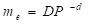(30)

Expressions for permeability and flow path length become,

KH = B(m-me)b and L = C(m-me)c (31)

so that in the Darcy equation (Eq. 8),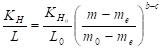(32)

where me is the equilibrium moisture ratio defined as that reached at the peak pressure after infinite time

Incorporating this into the DPM gives (Kerekes et al. 2014; McDonald et al. 2014a; McDonald and Kerekes 2017):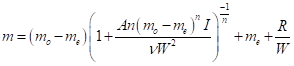(33)

Equation 33 provides a comprehensive predictor of wet pressing over the entire range of moisture content. In recent work it was employed to predict the upper limits of wet pressing on operating paper machines (McDonald and Kerekes 2017).

Equally useful, it quantifies Wahlstrom’s qualitative characterization of pressing regimes as “flow controlled” or “pressure controlled”. These regimes are illustrated in Figs. 3, 4 and 5 showing data, the qualitative picture, and the DP equation. .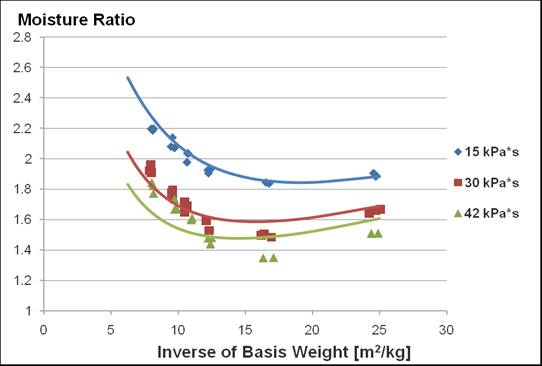Fig. 3. Moisture ratio after the nip in a pilot press for three different press impulses. Lines are fits to the DP model (McDonald et al. 2014a).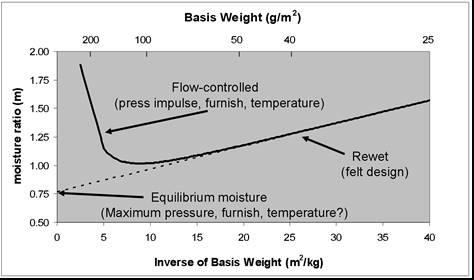Fig. 4. Wahlstrom Model

They can be seen to evolve from one regime to the other as basis weight is increased. Each term in the DP model equation corresponds to specific regions in Fig. 5.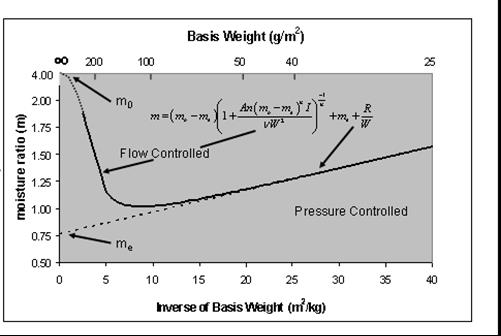Fig. 5. Wahlstrom Model with corresponding terms from the DP Model

SUMMARY AND CONCLUSIONS

This paper has evaluated pragmatic mathematical models to estimate moisture content of paper after wet pressing on paper machines. The selected models comprise a fraction of published wet pressing models, most of which are too complex for practical use. Most are also based on the concept of pressure being split between a hydraulic pressure causing water removal and a structural pressure supported by fibres. This concept implies that equilibrium is reached when there is no longer any pressure acting upon water. Given the large amount of water remaining in wet webs after pressing, the flexible and collapsible nature of fibres, and the short time of pressing, this approach has been found to be inconsistent with data from realistic experiments. In contrast, the pragmatic models discussed in this paper assume that all pressure acts on water whether directly or through fibre walls.

Press dewatering is a non-linear process that depends on many interacting factors. A pragmatic model must take into account all the major variables simultaneously. The Decreasing Permeability (DP) model is the only model developed thus far that meets this need. It permits estimates of moisture content after pressing using furnish-dependent coefficients determined from commercial pressing data or pilot press experiments. It also quantifies the “conventional picture” of flow-controlled and pressure-controlled regimes of wet pressing, along with rewet. As an engineering tool, the DP model can be used to evaluate optimization strategies that involve higher temperatures and press loadings, grade development, and new designs for shoes and felts. Future efforts will no doubt lead to improved models, but significant progress will only be likely if the models are built on a sound conceptual basis.

ACKNOWLEDGEMENTS

The authors would like to thank Ivan Pikulik and Ron Crotogino for useful comments and Ron Crotogino for help in translating Gudehus’ papers from German to English.

REFERENCES CITED

Bear, J. (1988). Dynamics of Fluids in Porous Media, Dover.

Bežanović, D., van Duijn, C. J., and Kaasschieter, E. F. (2006). “Analysis of paper pressing: The saturated one‐dimensional case,” ZAMM‐Journal of Applied Mathematics and Mechanics/Zeitschrift für Angewandte Mathematik und Mechanik 86(1), 18-36. DOI: 10.1002/zamm.200410220

Bezanovic, D., Van Duin, C. J., and Kaasschieter, E. F. (2007). “Analysis of wet pressing of paper: The three phase model, Part II: Compressible air case,” Transport in Porous Media. 67, 171-187. DOI: 10.1007/s11242-006-0018-8

Busker, L. H., and Cronin, D. C. (1984). “The relative importance of wet press variables in water removal: Effect of grammage,” Pulp and Paper Canada 85(6), 87-101.

Campbell, W. B. (1947). “The physics of water removal,” Pulp and Paper Magazine of Canada, 48(3), 103-109.

Carlsson, G., Lindstrom, T., and Soremark, C. (1977). “Expression of water from cellulosic fibres under compressive loading,” Trans., British Paper and Board Industry Federation Symposium, Fibre-Water Interactions in Papermaking, Oxford, p. 389-409.

Carlsson, G., Lindstrom, T., and Norman, B. (1983). “Some basic aspects on wet pressing of paper,” Pulp Paper Can. 84(9), R101-R106.

Caulfield, D. F., Young, T. L., and Wegner, T. H. (1986). “How web and press parameters interact to control water removal in the wet press,” Tappi J. 69(6), 90-93.

Caulfield, D. F., Young, T. L., and Wegner, T. H. (1982). “The role of web properties in water removal by wet pressing,” Tappi 65(2), 65-69.

Clos, R. J., Edwards, L. L., and Gunawan, I. (1994). “A limiting-consistency model for pulp dewatering and wet pressing,” Tappi J. 77(6), 179-187.

Cox, C. J., and Robertson, G. J. (1981). “The KMW press simulator – A tool for pressing studies,” Appita 35(1), 29-34.

DeCrosta, E. F., and Plaisted, W. E. (1978). “From the laboratory to the paper mill,” Tappi 61(9), 29-32.

DeCrosta, E. F. (1982). “Toward a unified theory of pressing,” Pulp & Paper Canada 83(1), 65-68.

Dunford, J. A., and Wild, P. M. (2002). “Cyclic transverse compression of single wood-pulp fibres,” Journal of Pulp and Paper Science 28(4), 136-141.

El-Hosseiny, F. (1991). “Mathematical modeling of wet paper – A review of the literature,” Nordic Pulp and Paper Research Journal 6(1), 30-34. DOI: 10.3183/NPPRJ-1991-06-01-p030-034

Fitt, A. D., Howell, P. D., King, J. R., Please, C. P., and Schwendeman, D. W. (2002). “Multiphase flow in a roll press nip,” European Journal of Applied Mathematics 13(3), 225-259. DOI: 10.1017/S0956792501004636

Gudehus, T. (1988a). “Stoffentwässerung im Walzenpressspalt,” Das Papier 42(4), 174-184.

Gudehus, T. (1988b). “Stoffentwässerung im Walzenpressspalt: Entwasserungsleistung Teil II,” Das Papier 42(5), 221-233.

Gudehus, T. (1988c). “Stoffentwässerung im Walzenpressspalt: Einflusfaktoren Teil III,” Das Papier 42(7), 361-375.

Gullbrand, J., and Vomhoff, H. (2005). “The influence of press felt micro-scale stress variation on dewatering,” Nordic Pulp and Paper Research Journal 20(3), 289-297. DOI: 10.3183/NPPRJ-2005-20-03-p289-297

Gustafsson, J. E., Kaul, V., Lobosco, V., and Bloch, J. F. (2003). “Modélisation du pressage du papier: Consolidation et expression de l’eau du gâteau fibreux humide,” ATIP. Association Technique de l’Industrie Papetière 57(4), 22-35.

Holstege, G. H. J. (1998). “Press dewatering: New life for an old concept,” Tappi J. 81(6), 115-121.

Iliev, O., Printsypar, G., and Rief, S. ( 2012). “On mathematical modeling and simulation of the pressing section of a paper machine including dynamic capillary effects: One-dimensional model,” Transport in Porous Media. 92(1), 41-59. DOI: 10.1007/s11242-011-9890-y

Iliev, O., Printsypar, G., and Rief, S. (2013). “A two-dimensional model of the pressing section of a paper machine including dynamic capillary effects,” Journal of Engineering Mathematics 83(1), 81-107. DOI: 10.1007/s10665-012-9619-0

Jackson, G. W., and James, D. F. (1986). “The permeability of fibrous porous media,” The Canadian Journal of Chemical Engineering 64(3), 364-374. DOI: 10.1002/cjce.5450640302

Jönsson, K. A., and Jönsson, B. T. L. (1992a). “Fluid flow in compressible porous media: I: Steady-state conditions,” AIChE Journal 38(9), 1340-1348. DOI: 10.1002/aic.690380905

Jönsson, K. A., and Jönsson, B. T. L. (1992b). “Fluid flow in compressible porous media: II: Dynamic behavior,” AIChE Journal 38(9), 1349-1354. DOI: 10.1002/aic.690380905

Kataya, M., Hiltunen, K., and Tomonen, J. (1992). “Flow of water and air in a compressible porous medium: A model of wet pressing of paper,” J. Phys. D: Appl. Phys. 25, 1053-1063. DOI: 10.1088/0022-3727/25/7/004

Kataya, M., Kirmanen, J., and Timonen, J. (1995). “Hydrostatic and structural pressure in compressed paper webs and press felts,” Nordic Pulp Pap. Res. J. 10(3), 162-166. DOI: 10.3183/NPPRJ-1995-10-03-p162-166

Kataya. (2009). “Flow permeability of fibrous porous materials. Micro-tomography and numerical simulations,” in: Proceedings in the 14th Fundamental Research Symposium, pp. 437-454.

Kerekes, R. J., and McDonald, J. D. (1991). “A decreasing permeability model of wet pressing: Theory,” Tappi J. 74(12), 150-156.

Kerekes, R. J., McDonald, E. M., and McDonald, J. D. (2014). “Decreasing permeability model of wet pressing: Extension to equilibrium conditions,” J-FOR 3(2), 46-51.

Koivu, V., Decain, M., Geindreau, C., Mattila, K., Bloch, J. F., and Kataja, M., (2009a). “Transport properties of heterogeneous materials. Combining computerised X-ray micro-tomography and direct numerical simulations,” International Journal of Computational Fluid Dynamics 23(10), 713-721. DOI: 10.1080/10618561003727512

Koivu, V., Decain, M., Geindreau, C., Mattila, K., Alaraudanjoki, J., Bloch, J. F., and Kataja, M. (2009b). “Flow permeability of fibrous porous materials. Micro-tomography and numerical simulations,” In Proceedings in the 14th Fundamental Research Symposium, pp. 437-454.

Koivu, V., Seppänen, R., Turpeinen, T., Mattila, K., Hyväluoma, J., and Kataja, M. (2010). “Combining X-ray micro-tomography and image analysis to study imbibition and void space in liquid packaging board,” Journal of Pulp and Paper Science 36(3-4), 170-177.

Laivins, G. V., and Scallan, A. M. (1994). “Removal of water from pulps by pressing. Inter-and intra-wall water,” Tappi J. 77(3), 125-131.

Lobosco, V. (2004). “On the modelling of mechanical dewatering in papermaking,” Doctoral Dissertation, Royal Institute of Technology, Division of Paper Technology, Stockholm.

Luey, A. T. (1979). “Pressing recycled paperboard,” EUCEPA Conference Proceedings, 124-133.

MacGregor, M. A. (1989). “Wet pressing research in 1989,” in: Transactions of the Ninth Fundamental Research Symposium, Cambridge, Mechanical Engineering Publications, Ltd., London UK, 511-586.

Maloney, T. C., Li, T. Q., Weise, U. L., and Paulapuro, H. A. (1997). “Intra-and inter-fibre pore closure in wet pressing,” Appita 50(4), 301-306.

McDonald, J. D., and Kerekes, R. J. (1991). “A decreasing permeability model of wet pressing: Applications,” Tappi J. 74(12), 142-149.

McDonald, J. D., and Kerekes, R. J. (1995). “A decreasing permeability model of wet pressing with rewetting,” Tappi J. 78(11), 107-111.

McDonald, J. D., Hamel, J., and Kerekes, R. J. (2000). “Design equation for paper machine press sections,” J. Pulp Pap. Sci. 26(11), 401-406.

McDonald, J. D., McDonald, E. M., and Kerekes, R. J. (2014a). “The impact of felt design on paper machine press dewatering,” J-FOR 3(2), 53-57.

McDonald, D., Ouellet, D., Tchepel, M., Wild, P., Provan, J., and Jeffrey, D. (2014b). Wood mechanics: From chips to flocs to fibres – Part II.” J-FOR 4(5), 31-37.

McDonald, J. D., and Kerekes, R. J. (2017). “Estimating limits of wet pressing on paper machines,” Tappi J. 16(2), 81-87.

Printsypar, G. (April 2012). “Mathematical modeling and simulation of two-phase flow in porous media with application to the pressing section of a paper machine,” Doctoral Dissertation, Technische Universitat Kaiserslautern, Fachbereich Mathematik.

Robertson, A. A., and Mason, S. G. (1949). “Specific surface of cellulose fibres by the liquid permeability method,” Pulp Paper Mag. Canada 50(13), 103-110.

Roux, J. C., and Vincent, J. P. (1991). “A proposed model in the analysis of wet pressing,” Tappi J. 74(2), 189-196.

Scallan, A. M. (1966). “The accommodation of water within pulp fibres,” in: Consolidation of the Paper Web, BPBIF Vol. 1, 9

Scheidegger, A. E. (1960). The Physics of Flow through Porous Media, 2nd Ed., University of Toronto Press, pp. 21-22, 112-138.

Tam Doo, P. A., and Kerekes, R. J. (1982). “The flexibility of wet pulp fibres,” Pulp and Paper Canada 83(2), 46-50.

Terzaghi, K., Peck, R. B., and Mesri, G. (1996). Soil Mechanics in Engineering Practice, John Wiley & Sons, pp. 83-84.

Vomhoff, H. (1998). Dynamic Compressibility of Water-Saturated Fibre Networks and the Influence of Local Stress Variations in Wet Pressing, Doctoral Thesis, Royal Institute of Technology, Department of Paper Technology, Stockholm

Wahlstrom, P. B. (1969). “Our present understanding of the fundamentals of pressing,” Pulp Paper Mag. Can. 70(19), 76-96

Wahlstrom, P. B. (1990). “Effect of water in the fibre wall on wet pressing,” in: Preprints, 44th APPITA Annual General Meeting, Rotoroa, A21.1-A21.24.

Wegner, T. H., Young, T. L., and Caulfield, D. F. (1983). “Role of fiber geometry in water removal by wet pressing,” Tappi 66(4), 85-88.

Young, T. L., Caulfield, D. F., and Wegner, T. H. (1983). “Role of web properties in water removal by wet pressing: influence of basis weight and forming method,” Tappi J. 66(10), 100-102.

Article submitted: July 6, 2017; Peer review completed: August 26, 2017; Revised version received and accepted: September 11, 2017; Published: September 15, 2017.

DOI: 10.15376/biores.12.4.McDonald